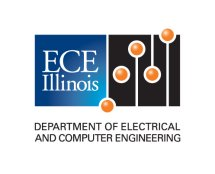ECE 453
Wireless Communication Systems

## Course Goals

The goals are to introduce students to circuits and systems employed for radio communication, and to provide an introduction to methods for analysis, design, and experimental measurement and characterization of communication circuits and systems.This course includes a laboratory section.

By the time of Exam No. 1 (after 19 lectures), the students should be able to do the following:
1. Identify and write mathematical expressions for carrier signals modulated using amplitude or angle modulation. (a)
2. Determine or estimate the bandwidth of a modulated carrier signal. (a)
3. Understand and analyze the operation of a superheterodyne receiver employing single or multiple frequency conversions. (a)
4. Design a superheterodyne receiver at the block-diagram level, including specification of required filter characteristics. (a,c)
5. Analyze an LC or quartz crystal oscillator circuit to determine conditions required for oscillation to start and to determine the frequency of oscillation. (a)
6. Design an LC or quartz crystal oscillator for operation at a specific frequency. (a,c)
7. Design a matching network using 2 lossless elements to match complex source and load impedances. (a,c)
8. Design a matching network using 3 lossless elements to match real source and load impedances with specified bandwidth. (a,c)
9. Design a matching network consisting of cascaded L-sections to match real source and load impedances with specified bandwidth. (a,c)
10. Measure the complex impedance of components at high frequencies using a Vector Network Analyzer. (a,b)
11. Accurately model the characteristics of passive components at high frequencies. (a)
12. Use a Spectrum Analyzer to characterize a radio frequency signal. (a,b)
13. By the time of Exam No. 2 (after 36 lectures), the students should be able to do all of the items listed under A, plus the following:
14. Understand the scattering parameter description of a linear 2-port. (a)
15. Measure the scattering parameters of a linear 2-port using a Vector Network Analyzer. (a,b)
16. Use scattering parameters of a 2-port to predict the input and output impedance, and power transfer between arbitrary complex source and load impedances. (a)
17. Determine if a 2-port is unconditionally stable, or not.If not, predict what source and load impedances can lead to instability. (a)
18. Design a small signal linear amplifier with conjugately matched input and output ports using scattering parameters. (a,c)
19. Understand the properties of a lossless filter network in terms of the scattering parameters. (a)
20. Understand the Butterworth, Chebyshev, Bessel low-pass filter approximations. (a)
21. Design a lossless low-pass filter using an LC ladder network and the Butterworth, Chebyshev, or Bessel approximation. (a,c)
22. Design a bandpass filter by transforming a low-pass prototype filter. (a,c)
23. Understand how to parameterize 2-port noise in terms of an effective input temperature. (a)
24. Apply Friis' formula to predict the effective input temperature of a cascade of noisy 2-ports. (a)
25. Calculate the effective input temperature of a passive, linear, lossy 2-port. (a)
26. Calculate the signal to noise ratio at the demodulator input given the effective noise temperature of the antenna and the 2-ports that comprise the receiver. (a)
27. Understand the Y-factor method for measuring the effective input temperature of a 2-port, including the influence of measurement inaccuracies. (a)
28. Measure the Noise Figure of a 2-port using an automated Noise Figure Meter. (a,b)
29. Understand the origin of intermodulation products in a nonlinear 2-port. (a)
30. Predict the frequencies of intermodulation products at the output of a nonlinear 2-port that result from multiple input tones. (a)
31. 30.Measure the 1 dB compression level and the two-tone third-order intercept level for a nonlinear 2-port. (a,b)
32. By the time of the Final Examination (after 41 lectures), the students should be able to do all of the items listed under A and B, plus the following:
33. Understand and analyze the operation of a switching mixer implemented using 3-winding transformers and diodes. (a)
34. Understand the basic operation of a Phase-Lock-Loop (PLL). (a)
35. Analyze the transient response of a PLL. (a)
36. Understand how PLL's are applied in modern communication systems. (a)
37. Design (at the block diagram level) a basic frequency synthesizer using a PLL. (c)